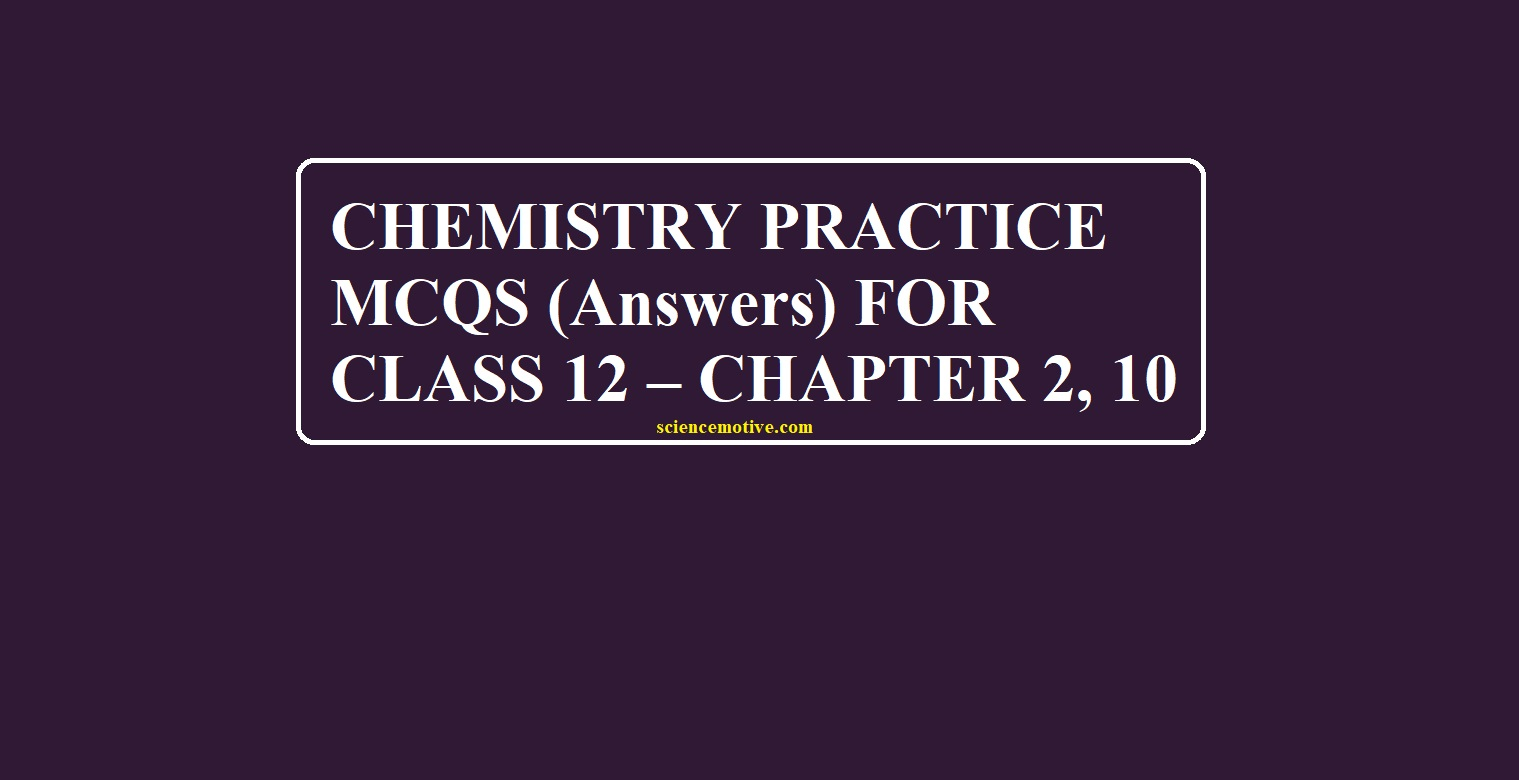# CHEMISTRY PRACTICE MCQS (Answers) FOR CLASS 12 – CHAPTER 2, 10To get questions for the post-Chemistry Practice MCQs (Answers) for Class 12 click the link at the end of the post

# Chemistry Practice MCQs (Answers) for Class 12

1. (d) 0.02

2. (d) 0.1 M Aluminium nitrate

3. (c) Boiling Point Increases and Freezing Point Decreases

4. (a) Urea

5. (d) Potassium sulphate

6. (c) Concentration of solute particles

7. (a) Osmotic pressure

8. (b) Flow of solvent particles, from solvent to solution through SPM

9. (a) Calcium chloride

10. (c) There is no flow of water

11. (a) Increasing temperature of the solution

12. (a) The enthalpy of mixing is zero

13. (a) Both A and R are true and R is the correct explanation of A

14. (c) A is true but R is false

15. (a) Both A and R are true and R is the correct explanation of A

16. Both assertion and reason are the wrong statements

17. (a) Both A and R are true and R is the correct explanation of A

18. (b) Both A and R are true but R is NOT the correct explanation of A

19. (a) Both A and R are true and R is the correct explanation of A

20. (i). (c) Boil above 100°C and freeze below 0°C

ii). (a) Dependent only on the concentration of the solute and independent of the solvents and solute’s identity.

iii). (d) All have the same freezing point.

iv). (d) All of The Above

21. (b) (iii) > (ii) > (i)

22. (d)

23. (c) (iii) < (i) < (ii)

24. (b) A substitution reaction

25. (d) (CH3)3C—I

26. (a) Cl2/UVlight

27. (b) (i), (ii), (iii)

28. (A)

29. (A)

30. (c)

31. (i) (d) (CH3)3C-I

(ii) (c) Inversion of configuration

(iii) (d) 1-Bromo-2-methylbutane

(iv) (a) SN1 mechanism

32. (c) ZnO + benzene

33. (a) NaHCO3 solution

Chemistry Practice MCQs (Answers) for Class 12

CHEMISTRY PRACTICE MCQS FOR CLASS 12 – CHAPTER 2, 10